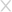## 快速检测恶意RTF文件的POC

2012-08-21 166631人围观 ，发现 1 个不明物体

CVE-2010-3333就是利用MicrosoftOffice RTF 分析器堆栈溢出漏洞，在网络大肆传播。国外安全爱好者Alexander Hanel日前发布了快速检测恶意RTF文件的POC。该POC利用了两种算法，据称识别率达到了97%，详见如下：

```# rtf-anom-scan.py
# This is a POC for detecting malicious RTF documents. The two algorithms are simple
# The first one counts the amount of non-ASCII data in a file and the second
# calculates the entropy of ASCII Hex blobs. Please see comments and code below for more details.
# These can be broken pretty easy but the script currently detects 97% of the .RTF samples on
# contagiodump.  Out of 169 random .RTFs found via Google and FTP searches there was 1 FP. The
# FP was caused possibly by Unicode text. There is no error handling. Just make sure the file is a
# .RTF and the script has read writes.
# Written by alexander.hanel@gmail.com
#
# usage:
# For scanning a RTF document "rtf-anom-scan.py <bad.rtf>"
# For scanning a working dir "rtf-anom-scan.py"

import sys
import os
import re
import string
import math

# Checks for the RTF header '\rt' in the file
# Non-RTF files will give false positives
f = open(fi,'rb')
if '\\rt' not in block:
print '\t',

return

def H(data):
# calculates the entropy of a block of data
# from Ero's blog http://blog.dkbza.org/2007/05/scanning-data-for-entropy-anomalies.html
if not data:
return 0
entropy = 0
for x in range(256):
p_x = float(data.count(chr(x)))/len(data)
if p_x > 0:
entropy += - p_x*math.log(p_x, 2)
return entropy

def shell_ent(fi):
index = 0
block_size = 128
tmp = 0
inc = 0
with open(fi,'rb') as f:
while(data != ''):
m = ''
# Search for blobs of data that are valid hex [a-fA-F0-9]
m = re.search(r'[a-fA-F0-9]{128}',data)
if m:
# ASCII HEX shellcode has consistent entropy between 3.6 and 4.0
# We can use the entropy to detect shellcode in files that do not
# contain non-ASCII values. Commonly seen in shellcode that does
entropy = H(data)
if 4.0 > entropy > 3.6:
if tmp == index - 16:
inc = inc + 1
if inc == 16:
print "Suspicious: shellcode entropy block at %s in %s" % (hex(index),fi)
return
#print hex(index), entropy, inc
else:
inc = 0

f.seek(index)
tmp = index
index = index + 16

return

def valid_ascii(char):
# Check if valid ASCII
if char in string.printable[:-3] + '\x0d':
return True
else:
return None

def check_bytes(file_):
# Counts the amount of non-ASCII bytes are in a file
count = 0
with open(file_,'rb') as f:
while byte != '':
if valid_ascii(byte) == None:
count = count + 1
if count > 10000:
print "Suspicious: large amounts of non-ASCII chars %s" % file_
return True
return False

def main():
if len(sys.argv) == 2:
if check_bytes(sys.argv) != True:
shell_ent(sys.argv)
else:
for infile in os.listdir(os.getcwd()):
if check_bytes(infile) != True:
shell_ent(infile)

if __name__ == '__main__':
main()```### 相关阅读

文章目录

推荐关注官方公众号聚焦企业安全

### 活动预告### 填写个人信息

• 可以给我们打个分吗？